The SURVEYFREQ Procedure

Confidence Limits for Proportions

If you specify the CL option in the TABLES statement, PROC SURVEYFREQ computes confidence limits for the proportions in the frequency and crosstabulation tables.

By default, PROC SURVEYFREQ computes Wald (linear) confidence limits if you do not specify an alternative confidence limit type with the CL(TYPE=) option. In addition to Wald confidence limits, the following types of design-based confidence limits are available for proportions: modified Clopper-Pearson (exact), modified Wilson (score), and logit confidence limits.

PROC SURVEYFREQ also provides the CL(PSMALL) option, which uses the alternative confidence limit type for extreme (small or large) proportions and uses the Wald confidence limits for all other proportions (not extreme). For the default PSMALL= value of 0.25, the procedure computes Wald confidence limits for proportions between 0.25 and 0.75 and computes the alternative confidence limit type for proportions that are outside of this range. See Curtin et al. (2006).

For details about confidence limits for proportions based on complex survey data, including comparisons of their performance, see Korn and Graubard (1999, 1998); Curtin et al. (2006); Sukasih and Jang (2005). Also, in addition to the other references cited in the following sections, for information about binomial confidence limits see Brown, Cai, and DasGupta (2001); Agresti and Coull (1998).

For each table request, PROC SURVEYFREQ produces a nondisplayed ODS table, Table Summary, which contains the number of observations, strata, and clusters that are included in the analysis of the requested table. When you request confidence limits, the Table Summary data set also contains the degrees of freedom df and the value of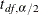that is used to compute the confidence limits. See Example 90.3 for more information about this output data set.

Wald Confidence Limits

PROC SURVEYFREQ computes standard Wald (linear) confidence limits for proportions by default. These confidence limits use the variance estimates that are based on the sample design. For the proportion in table cell (r, c), the Wald confidence limits are computed as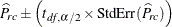where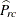is the estimate of the proportion in table cell (r, c),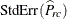is the standard error of the estimate, andis the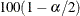percentile of the t distribution with df degrees of freedom calculated as described in the section Degrees of Freedom. The confidence levelis determined by the value of the ALPHA= option, which by default equals 0.05 and produces 95% confidence limits.

The confidence limits for row proportions and column proportions are computed similarly to the confidence limits for table cell proportions.

Modified Confidence Limits

PROC SURVEYFREQ uses the modification described in Korn and Graubard (1998) to compute design-based Clopper-Pearson (exact) and Wilson (score) confidence limits. This modification substitutes the degrees-of-freedom adjusted effective sample size for the original sample size in the confidence limit computations.

The effective sample size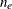is computed as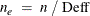where n is the original sample size (unweighted frequency) that corresponds to the total domain of the proportion estimate, and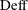is the design effect.

If the proportion is computed for a table cell of a two-way table, then the domain is the two-way table, and the sample size n is the frequency of the two-way table. If the proportion is a row proportion, which is based on a two-way table row, then the domain is the row, and the sample size n is the frequency of the row.

The design effect for an estimate is the ratio of the actual variance (estimated based on the sample design) to the variance of a simple random sample with the same number of observations. See the section Design Effect for details about how PROC SURVEYFREQ computes the design effect.

If you do not specify the CL(ADJUST=NO) option, the procedure applies a degrees-of-freedom adjustment to the effective sample size to compute the modified sample size. If you specify CL(ADJUST=NO), the procedure does not apply the adjustment and uses the effective sample sizein the confidence limit computations.

The modified sample size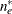is computed by applying a degrees-of-freedom adjustment to the effective sample sizeas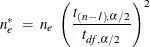where df is the degrees of freedom andis thepercentile of the t distribution with df degrees of freedom. The section Degrees of Freedom describes the computation of the degrees of freedom df, which is based on the variance estimation method and the sample design. The confidence levelis determined by the value of the ALPHA= option, which by default equals 0.05 and produces 95% confidence limits.

The design effect is usually greater than 1 for complex survey designs, and in that case the effective sample size is less than the actual sample size. If the adjusted effective sample sizeis greater than the actual sample size n, then the procedure truncates the value ofto n, as recommended by Korn and Graubard (1998). If you specify the CL(TRUNCATE=NO) option, the procedure does not truncate the value of.

Modified Clopper-Pearson Confidence Limits

Clopper-Pearson (exact) confidence limits for the binomial proportion are constructed by inverting the equal-tailed test based on the binomial distribution. This method is attributed to Clopper and Pearson (1934). See Leemis and Trivedi (1996) for a derivation of the F distribution expression for the confidence limits.

PROC SURVEYFREQ computes modified Clopper-Pearson confidence limits according to the approach of Korn and Graubard (1998). The degrees-of-freedom adjusted effective sample sizeis substituted for the sample size in the Clopper-Pearson computation, and the adjusted effective sample size times the proportion estimate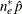is substituted for the number of positive responses. (Or if you specify the CL(ADJUST=NO) option, the procedure uses the unadjusted effective sample sizeinstead of.)

The modified Clopper-Pearson confidence limits for a proportion (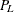and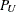) are computed as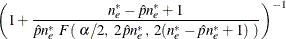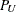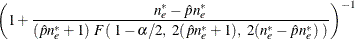where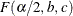is the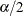percentile of the F distribution with b and c degrees of freedom,is the adjusted effective sample size, and
is the proportion estimate.

Modified Wilson Confidence Limits

Wilson confidence limits for the binomial proportion are also known as score confidence limits and are attributed to Wilson (1927). The confidence limits are based on inverting the normal test that uses the null proportion in the variance (the score test). See Newcombe (1998) and Korn and Graubard (1999) for details.

PROC SURVEYFREQ computes modified Wilson confidence limits by substituting the degrees-of-freedom adjusted effective sample sizefor the original sample size in the standard Wilson computation. (Or if you specify the CL(ADJUST=NO) option, the procedure substitutes the unadjusted effective sample size.)

The modified Wilson confidence limits for a proportion are computed as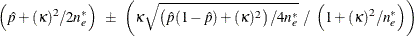whereis the adjusted effective sample size andis the estimate of the proportion. With the degrees-of-freedom adjusted effective sample size, the computation uses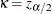. With the unadjusted effective sample size, which you request with the ADJUST=NO option, the computation uses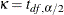. See Curtin et al. (2006) for details.

Logit Confidence Limits

If you specify the CL(TYPE=LOGIT) option, PROC SURVEYFREQ computes logit confidence limits for proportions. See Agresti (2002) and Korn and Graubard (1998) for more information.

Logit confidence limits for proportions are based on the logit transformation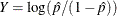. The logit confidence limitsandare computed as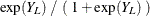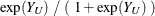where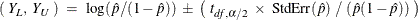whereis the estimate of the proportion,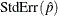is the standard error of the estimate, andis thepercentile of the t distribution with df degrees of freedom. The degrees of freedom are calculated as described in the section Degrees of Freedom. The confidence levelis determined by the value of the ALPHA= option, which by default equals 0.05 and produces 95% confidence limits.